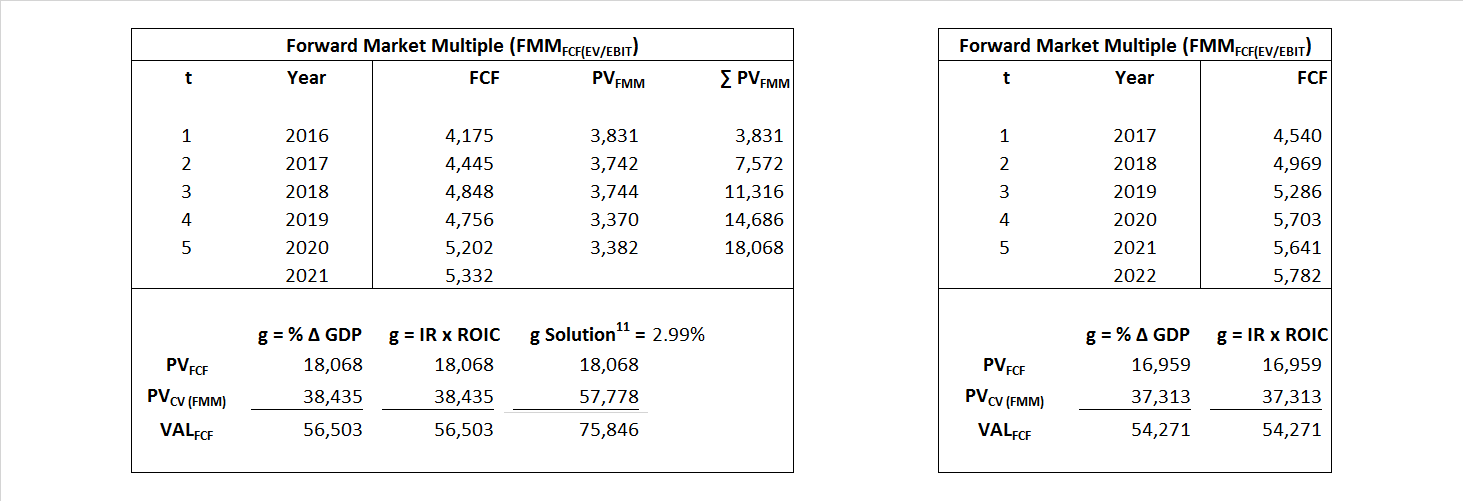# Time Warner

## Analyst Listing

The following analysts provide coverage for the subject firm as of May 2016:

 Pacific Crest Securities-KBCM Andy Hargreaves ahargreaves@pacific-crest.com Nomura Anthony DiClemente anthony.diclemente@nomura.com Stifel Nicolaus Benjamin E. Mogil bmogil@stifel.com Pivotal Research Group Brian Wieser brian@pvtl.com Deutsche Bank Research Bryan Kraft bryan.kraft@db.com Topeka Capital Markets David W. Miller dwm@topekacapitalmarkets.com Cowen & Company Doug Creutz doug.creutz@cowen.com Wells Fargo Securities John Janedis jjanedis@jefferies.com Needham Laura Martin lmartin@needhamco.com Cowen & Company Marci Ryvicker marci.ryvicker@wellsfargo.com Guggenheim Securities Michael C. Morris michael.morris@guggenheimpartners.com RBC Capital Markets Steven Cahall steven.cahall@rbccm.com Drexel Hamilton Tony Wible twible@drexelhamilton.com Deutsche Bank Research Bryan Kraft bryan.kraft@db.com Raymond James Frank G. Louthan IV frank.louthan@raymondjames.com Barrington Research James C. Goss jcg@brai.com Scotiabank GBM Jeffrey Fan jeff.fan@scotiabank.com RBC Capital Markets Jonathan Atkin jonathan.atkin@rbccm.com Needham Laura Martin lmartin@needhamco.com Wells Fargo Securities Marci Ryvicker marci.ryvicker@wellsfargo.com Wunderlich Securities Matthew Harrigan mharrigan@wundernet.com Jefferies Mike McCormack mmccormack@jefferies.com Evercore ISI Vijay Jayant vijay.jayant@evercoreisi.com

## Primary Input Data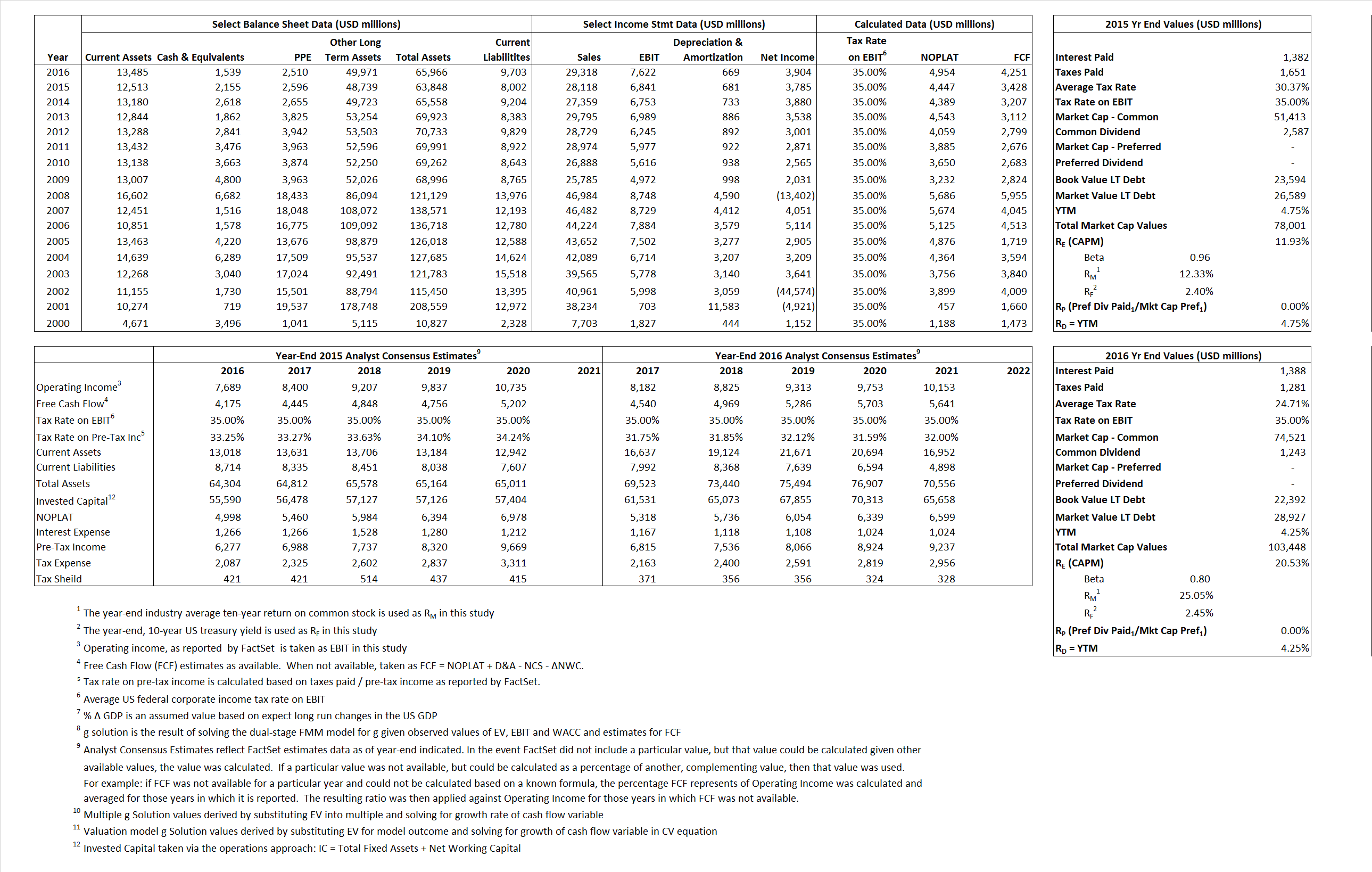## Derived Input Data

### Equational Form

Net Operating Profit Less Adjusted Taxes NOPLAT   4,447  4,954$NOPLAT\, =\, EBIT\, x\, (1 \,-\, Avg \,\,Tax\,\, Rate\,\, on\,\, EBIT)$
Free Cash Flow FCF  3,428  4,251$FCF\,=NOPLAT\,+\,Non-Cash\,Expenses-\Delta NWC\,-\,NCS$
Tax Shield TS  420  343$TS\,=\,Interest\,\,Paid\,\,x\,\, Avg \,\,Tax\,\,Rate\,\, on\,\, Pre-Tax\,\, Income$
Invested Capital IC  55,846  56,263$IC\,=\,Fixed\,\,Operating\,\,Assets\,\,+\,\,Net\,\, Working\,\, Capital$
Return on Invested Capital ROIC 7.96% 8.81%$ROIC\,=\,\frac { NOPLAT }{ IC }$
Net Investment NetInv  173  1,086$NetInv\,=\,{ {IC}_{1}}-{{IC}_{0}}+Depreciation$
Investment Rate IR 3.89% 21.92%$IR\,=\,\frac {NetInv}{NOPLAT}$
Weighted Average Cost of Capital WACCMarket 8.99% 15.68%$WACC\,=\,\frac { E }{ V } { R }_{ E }\,+\,\frac { P }{ V } { R }_{ P }\,+\,\frac { D }{ V } { R }_{ D }\left( 1- Avg\,\, Tax\,\,Rate\,\,on\,\,Pre-Tax\,\,Income \right)$
WACCBook  6.95% 6.40%
Enterprise value EVMarket  75,846  101,909$EV\,=\,Market\,\,Cap\,\,Equity\,+\,\,Long\,\,Term\,\,Debt\,-\,Cash$
EVBook  73,468 95,374
EV/EBIT Multiple$\frac{EV_{Market}}{EBIT}$  11.09   13.37$EV/EBIT\,=\,\frac { EV}{ EBIT}$
Long-Run Growth g = IR x ROIC
0.31%    1.93% Long-run growth rates of the income variable  are used in the Continuing Value portion of the valuation models.
g = %$\Delta$ GDP   2.50%   2.50%

## Valuation Model Outcomes

The outcomes presented in this study are the result of original input data, derived data, and synthesized inputs and, depending on the equational form of any particular valuation model, may result in irrelevant or implausible results.  For example, in the event WACC < g, the value of this term, often found in the denominator of an equation’s continuation value term, will be expressly negative and may result in a negative overall valuation for the firm.  In the event of a WACC < g relation, the model form as applied to the subject firm offers an irrelevant outcome.

### Equational form

Key Value Driver (NOPLAT) KVD (NOPLAT)${ Value }_{ DCF/KVD }=\sum { \frac { NOPLAT_{ t } }{ { \left( 1+WACC \right) }^{ t } } +\frac { \frac { { NOPLAT }_{ 1 }\left( 1-\frac { g }{ ROIC } \right) }{ WACC-g } }{ { \left( 1+WACC \right) }^{ t } } }$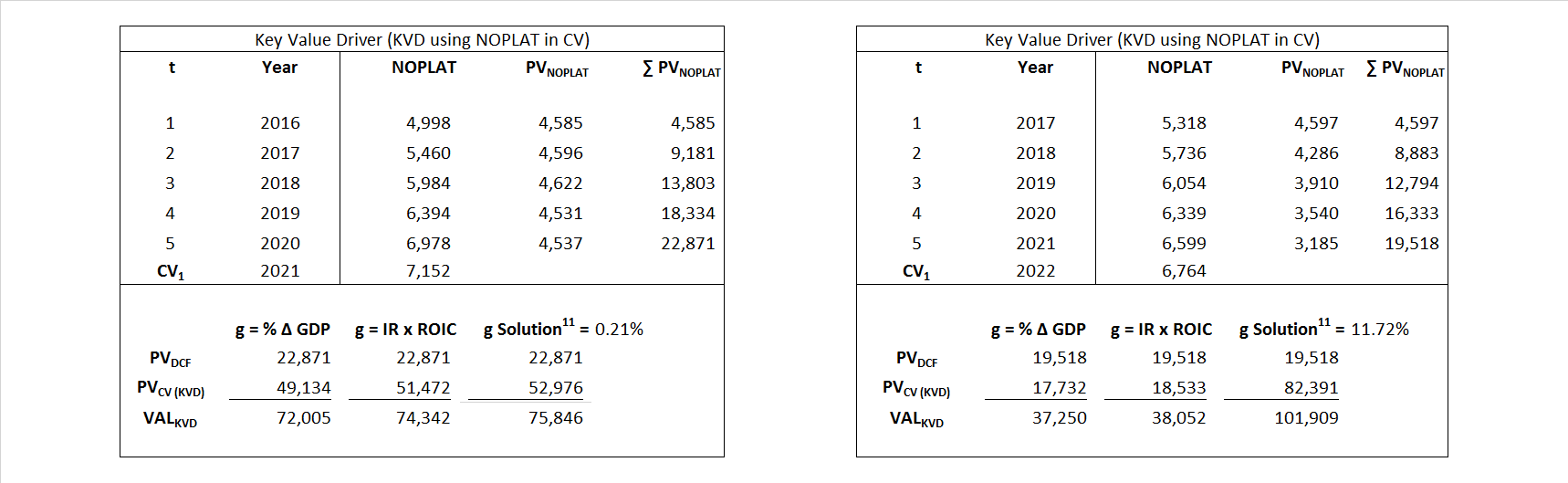Key Value Driver (FCF) KVD (FCF)${ Value }_{ DCF/KVD }=\sum { \frac { FCF_{ t } }{ { \left( 1+WACC \right) }^{ t } } +\frac { \frac { { NOPLAT }_{ 1 }\left( 1-\frac { g }{ ROIC } \right) }{ WACC-g } }{ { \left( 1+WACC \right) }^{ t } } }$Free Cash Flow FCF${ Value }_{ DCF/FCF }=\sum { \frac { FCF_{ t } }{ { \left( 1+WACC \right) }^{ t } } +\frac { \frac { { FCF }_{ 1 }}{ WACC-g } }{ { \left( 1+WACC \right) }^{ t } } }$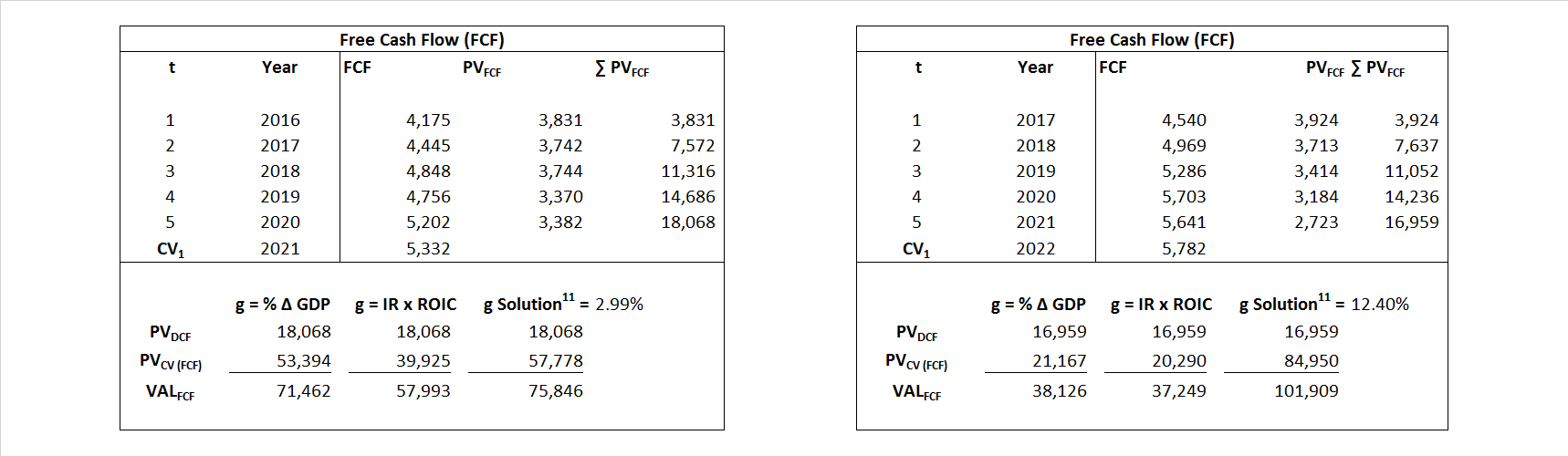Economic Profit ECON π${ Value }_{ { ECON\pi } }= I{ C }_{ 0 }+\sum { \frac { { IC }_{ t-1 }(ROI{ C }_{t}-WAC{C}_{t}) }{ { \left( 1+WACC \right) }^{ t } }+ \frac {\frac { I{C}_{0}\ x\ (ROI{C}_{1}\ -\ WAC{C}_{1}) }{ WACC-g } }{ { \left( 1+WACC \right) }^{ t } } }$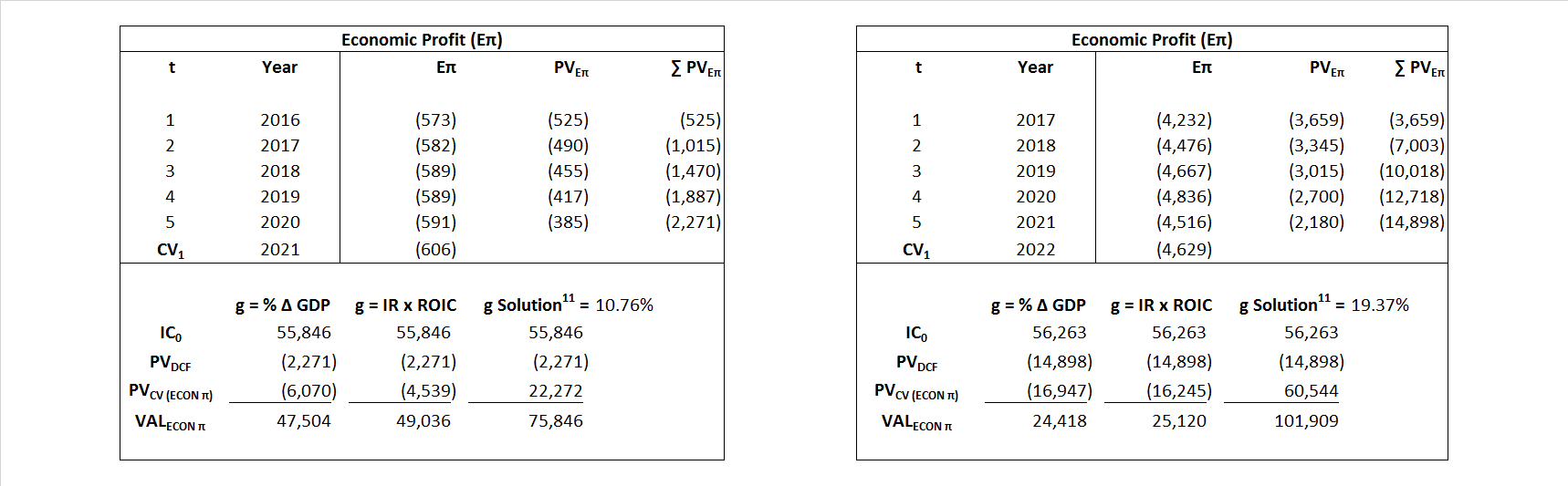Adjusted Present Value APV${ Value }_{ APV }=\sum { \frac { FCF_{ t } }{ { \left( 1+{ k }_{ u } \right) }^{ t } } +\frac { \frac { { FCF }_{ 1 }}{ { k }_{ u }-g } }{ { \left( 1+{ k }_{ u } \right) }^{ t } } } +\sum { \frac { { TS }_{ t } }{ { \left( 1+{ k }_{ tax } \right) }^{ t } } +\frac { \frac { { TS }_{ 1 }}{ { k }_{ tax }-g } }{ { \left( 1+{ k }_{ tax } \right) }^{ t } } }$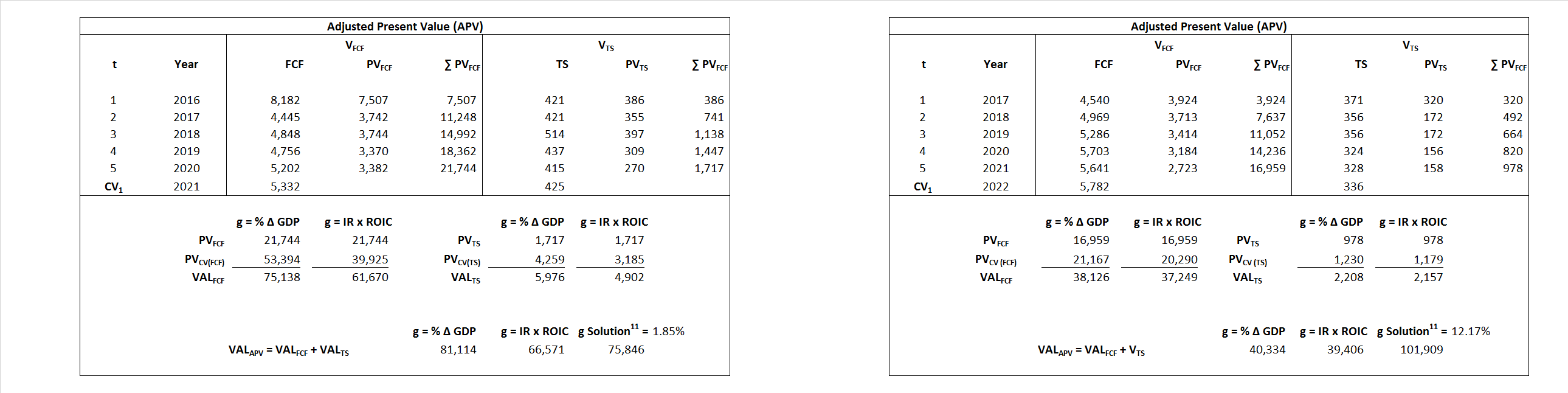Forward Market Multiple FMM${ Value }_{ DCF/FMM}=\sum { \frac { FCF_{ t } }{ { \left( 1+WACC \right) }^{ t } } +\frac { { EBIT }_{ 1 }\,{x}\,{FMM}}{ { \left( 1+WACC \right) }^{ t } } }{\,\,\,; \,\,FMM\,=\,\frac{{EV}_{t=0}}{{EBIT}_{t=0}}}$